# Dickman-function(2)

(diff) ← Older revision | Latest revision (diff) | Newer revision → (diff)

The unique continuous solution of the systemThe Dickman function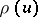occurs in the problem of estimating the number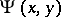of positive integers not exceedingthat are free of prime factors greater than: for any fixed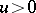, one hasas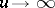[a2], [a4].

The functionis positive, non-increasing and tends to zero at a rate faster than exponential as. A precise asymptotic estimate is given by the de Bruijn–Alladi formula [a1], [a3]: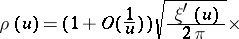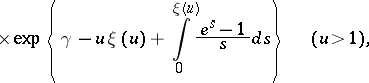whereis the Euler constant andis the unique positive solution of the equation.

How to Cite This Entry:
Dickman-function(2). Encyclopedia of Mathematics. URL: http://encyclopediaofmath.org/index.php?title=Dickman-function(2)&oldid=15579
This article was adapted from an original article by A. Hildebrand (originator), which appeared in Encyclopedia of Mathematics - ISBN 1402006098. See original article